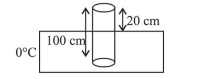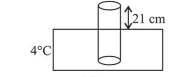# A leak proof cylinder of length 1 m,Question:

A leak proof cylinder of length $1 \mathrm{~m}$, made of a metal which has very low coefficient of expansion is floating vertically in water at $0^{\circ} \mathrm{C}$ such that its height above the water surface is $20 \mathrm{~cm}$. When the temperature of water is increased to $4^{\circ} \mathrm{C}$, the height of the cylinder above the water surface becomes $21 \mathrm{~cm}$. The density of water at $T=4^{\circ} \mathrm{C}$, relative to the density at $T=0^{\circ} \mathrm{C}$ is close to:

1. (1) $1.26$

2. (2) $1.04$

3. (3) $1.01$

4. (4) $1.03$

Correct Option: , 3

Solution:

(3) When cylinder is floating in water at $0^{\circ} \mathrm{C}$

Net thrust $=A\left(h_{2}-h_{1}\right) \rho_{0^{\circ}} \mathrm{c} g$

$=A(100-80) \rho_{0^{\circ} c} g$When cylinder is floating in water at $4^{\circ} \mathrm{C}$

Net thrust $=A\left(h_{2}-h_{1}\right) \rho_{4^{\circ} c} g$

$=A(100-21) \rho_{4^{\circ} c} g$$\therefore \frac{\rho_{4}{ }^{\circ} \mathrm{C}}{\rho_{0^{\circ} \mathrm{C}}}=\frac{80}{79}=1.01$